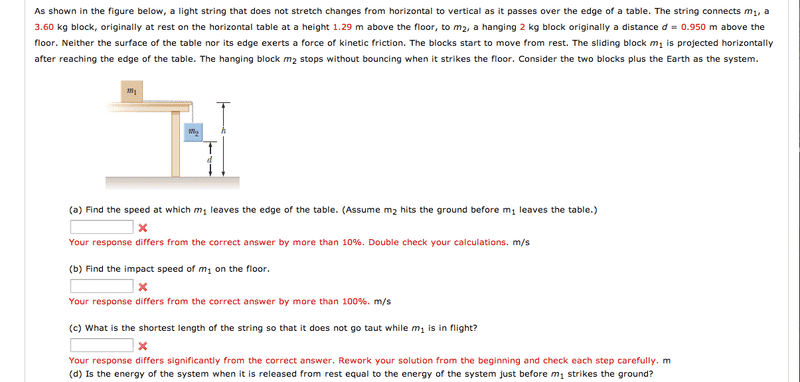# Conservation of Energy Problem

ianmgull

## Homework Statement## Homework Equations

ΔK1+ΔK2+ΔU1+ΔU2 = 0

## The Attempt at a Solution

The biggest stumbling block I have is setting up the initial and final conditions. First the initial:

1)Does m1 have potential energy in this setup? Part of me thinks it would have m1gh but I could also see it having zero potential energy (being on the table top).

2)I assume the final state would be right when m1 hits the ground (m2 has already hit). If this is the case, don't I need information at some intermediate state between the two? For instance, when m1 leaves the table?

Thanks.

## Answers and Replies

nil1996
Why don't you try applying newtons laws and kinematics and then energy principles?

Last edited:
ianmgull
I figured I would need to know the distance that m2 is from the edge of the table for that (which I don't know). Is there something I'm missing that you could point out?

nil1996
oh sorry first of all welcome to Physics forum :)
See that the mass m1 will experience force till mass m2 has not reached the ground. So mass m1 will keep on accelerating till mass m2 reaches the ground. So you can calculate how much it will accelerate as you know the distance of m2 from ground.Understood?

Last edited:
nasu
You can use either conservation of energy or the laws of motion.
I understand that you are expected to use energy laws, right?

The values of potential energy will depend on where do you choose the reference level.
If you use the floor, m1 will have potential energy (m1*g*h) in the initial state. And it will have the same potential energy in the final state (for part a).